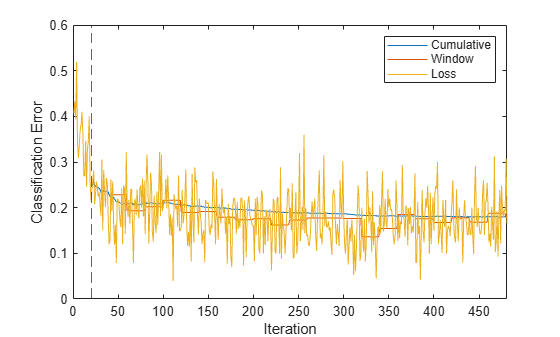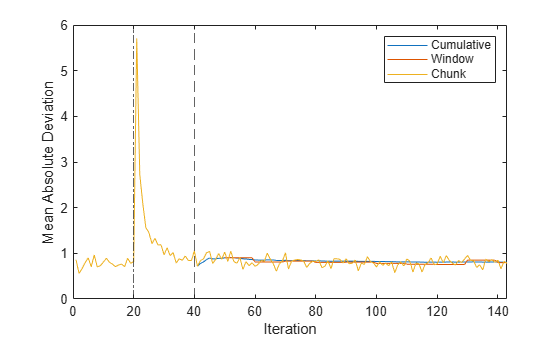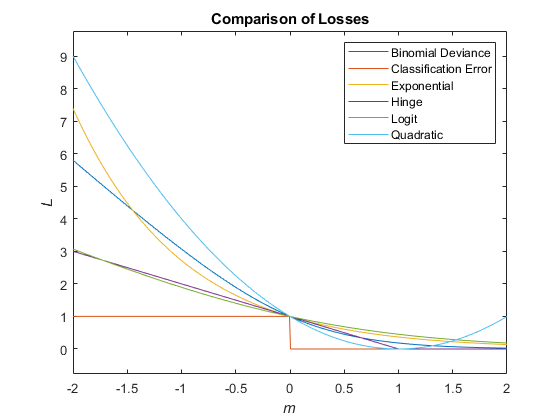# loss

Loss of kernel incremental learning model on batch of data

Since R2022a

## Syntax

``L = loss(Mdl,X,Y)``
``L = loss(Mdl,X,Y,Name=Value)``

## Description

`loss` returns the regression or classification loss of a configured incremental learning model for kernel regression (`incrementalRegressionKernel` object) or binary kernel classification (`incrementalClassificationKernel` object).

To measure model performance on a data stream and store the results in the output model, call `updateMetrics` or `updateMetricsAndFit`.

example

````L = loss(Mdl,X,Y)` returns the loss for the incremental learning model `Mdl` using the batch of predictor data `X` and corresponding responses `Y`.```

example

````L = loss(Mdl,X,Y,Name=Value)` uses additional options specified by one or more name-value arguments. For example, you can specify the classification loss function and the observation weights.```

## Examples

collapse all

The performance of an incremental model on streaming data is measured in three ways:

1. Cumulative metrics measure the performance since the start of incremental learning.

2. Window metrics measure the performance on a specified window of observations. The metrics are updated every time the model processes the specified window.

3. The `loss` function measures the performance on a specified batch of data only.

Load the human activity data set. Randomly shuffle the data.

```load humanactivity n = numel(actid); rng(1) % For reproducibility idx = randsample(n,n); X = feat(idx,:); Y = actid(idx);```

For details on the data set, enter `Description` at the command line.

Responses can be one of five classes: Sitting, Standing, Walking, Running, or Dancing. Dichotomize the response by identifying whether the subject is moving (`actid` > 2).

`Y = Y > 2;`

Create an incremental kernel model for binary classification. Specify a metrics window size of 1000 observations. Configure the model for `loss` by fitting it to the first 10 observations.

```p = size(X,2); Mdl = incrementalClassificationKernel(MetricsWindowSize=1000); initobs = 10; Mdl = fit(Mdl,X(1:initobs,:),Y(1:initobs));```

`Mdl` is an `incrementalClassificationKernel` model. All its properties are read-only.

Simulate a data stream, and perform the following actions on each incoming chunk of 50 observations:

1. Call `updateMetrics` to measure the cumulative performance and the performance within a window of observations. Overwrite the previous incremental model with a new one to track performance metrics.

2. Call `loss` to measure the model performance on the incoming chunk.

3. Call `fit` to fit the model to the incoming chunk. Overwrite the previous incremental model with a new one fitted to the incoming observations.

4. Store all performance metrics to see how they evolve during incremental learning.

```% Preallocation numObsPerChunk = 50; nchunk = floor((n - initobs)/numObsPerChunk); ce = array2table(zeros(nchunk,3),VariableNames=["Cumulative","Window","Loss"]); % Incremental learning for j = 1:nchunk ibegin = min(n,numObsPerChunk*(j-1) + 1 + initobs); iend = min(n,numObsPerChunk*j + initobs); idx = ibegin:iend; Mdl = updateMetrics(Mdl,X(idx,:),Y(idx)); ce{j,["Cumulative","Window"]} = Mdl.Metrics{"ClassificationError",:}; ce{j,"Loss"} = loss(Mdl,X(idx,:),Y(idx)); Mdl = fit(Mdl,X(idx,:),Y(idx)); end```

`Mdl` is an `incrementalClassificationKernel` model object trained on all the data in the stream. During incremental learning and after the model is warmed up, `updateMetrics` checks the performance of the model on the incoming observations, and the `fit` function fits the model to those observations. `loss` is agnostic of the metrics warm-up period, so it measures the classification error for all iterations.

To see how the performance metrics evolve during training, plot them.

```plot(ce.Variables) xlim([0 nchunk]) ylabel("Classification Error") xline(Mdl.MetricsWarmupPeriod/numObsPerChunk,"--") legend(ce.Properties.VariableNames) xlabel("Iteration")```The yellow line represents the classification error on each incoming chunk of data. After the metrics warm-up period, `Mdl` tracks the cumulative and window metrics. The cumulative and batch losses converge as the `fit` function fits the incremental model to the incoming data.

Fit an incremental learning model for regression to streaming data, and compute the mean absolute deviation (MAD) on the incoming data batches.

Load the robot arm data set. Obtain the sample size `n` and the number of predictor variables `p`.

```load robotarm n = numel(ytrain); p = size(Xtrain,2);```

For details on the data set, enter `Description` at the command line.

Create an incremental kernel model for regression. Configure the model as follows:

• Specify a metrics warm-up period of 1000 observations.

• Specify a metrics window size of 500 observations.

• Track the mean absolute deviation (MAD) to measure the performance of the model. Create an anonymous function that measures the absolute error of each new observation. Create a structure array containing the name `MeanAbsoluteError` and its corresponding function.

• Configure the model to predict responses by fitting it to the first 10 observations.

```maefcn = @(z,zfit,w)(abs(z - zfit)); maemetric = struct(MeanAbsoluteError=maefcn); Mdl = incrementalRegressionKernel(MetricsWarmupPeriod=1000,MetricsWindowSize=500, ... Metrics=maemetric); initobs = 10; Mdl = fit(Mdl,Xtrain(1:initobs,:),ytrain(1:initobs));```

`Mdl` is an `incrementalRegressionKernel` model object configured for incremental learning.

Perform incremental learning. At each iteration:

• Simulate a data stream by processing a chunk of 50 observations.

• Call `updateMetrics` to compute cumulative and window metrics on the incoming chunk of data. Overwrite the previous incremental model with a new one fitted to overwrite the previous metrics.

• Call `loss` to compute the MAD on the incoming chunk of data. Whereas the cumulative and window metrics require that custom losses return the loss for each observation, `loss` requires the loss on the entire chunk. Compute the mean of the absolute deviation.

• Call `fit` to fit the incremental model to the incoming chunk of data.

• Store the cumulative, window, and chunk metrics to see how they evolve during incremental learning.

```% Preallocation numObsPerChunk = 50; nchunk = floor((n - initobs)/numObsPerChunk); mae = array2table(zeros(nchunk,3),VariableNames=["Cumulative","Window","Chunk"]); % Incremental fitting for j = 1:nchunk ibegin = min(n,numObsPerChunk*(j-1) + 1 + initobs); iend = min(n,numObsPerChunk*j + initobs); idx = ibegin:iend; Mdl = updateMetrics(Mdl,Xtrain(idx,:),ytrain(idx)); mae{j,1:2} = Mdl.Metrics{"MeanAbsoluteError",:}; mae{j,3} = loss(Mdl,Xtrain(idx,:),ytrain(idx),LossFun=@(x,y,w)mean(maefcn(x,y,w))); Mdl = fit(Mdl,Xtrain(idx,:),ytrain(idx)); end```

`Mdl` is an `incrementalRegressionKernel` model object trained on all the data in the stream. During incremental learning and after the model is warmed up, `updateMetrics` checks the performance of the model on the incoming observations, and the `fit` function fits the model to those observations.

Plot the performance metrics to see how they evolved during incremental learning.

```plot(mae.Variables) ylabel("Mean Absolute Deviation") xlabel("Iteration") xlim([0 nchunk]) xline(Mdl.EstimationPeriod/numObsPerChunk,"-.") xline((Mdl.EstimationPeriod + Mdl.MetricsWarmupPeriod)/numObsPerChunk,"--") legend(mae.Properties.VariableNames)```The plot suggests the following:

• `updateMetrics` computes the performance metrics after the metrics warm-up period only.

• `updateMetrics` computes the cumulative metrics during each iteration.

• `updateMetrics` computes the window metrics after processing 500 observations (10 iterations).

• Because `Mdl` was configured to predict observations from the beginning of incremental learning, `loss` can compute the MAD on each incoming chunk of data.

## Input Arguments

collapse all

Incremental learning model, specified as an `incrementalClassificationKernel` or `incrementalRegressionKernel` model object. You can create `Mdl` directly or by converting a supported, traditionally trained machine learning model using the `incrementalLearner` function. For more details, see the corresponding reference page.

You must configure `Mdl` to predict labels for a batch of observations.

Batch of predictor data, specified as a floating-point matrix of n observations and `Mdl.NumPredictors` predictor variables.

The length of the observation labels `Y` and the number of observations in `X` must be equal; `Y(j)` is the label of observation j (row) in `X`.

Note

`loss` supports only floating-point input predictor data. If your input data includes categorical data, you must prepare an encoded version of the categorical data. Use `dummyvar` to convert each categorical variable to a numeric matrix of dummy variables. Then, concatenate all dummy variable matrices and any other numeric predictors. For more details, see Dummy Variables.

Data Types: `single` | `double`

Batch of responses (labels), specified as a categorical, character, or string array, a logical or floating-point vector, or a cell array of character vectors for classification problems; or a floating-point vector for regression problems.

The length of the observation labels `Y` and the number of observations in `X` must be equal; `Y(j)` is the label of observation j (row) in `X`.

For classification problems:

• `loss` supports binary classification only.

• If `Y` contains a label that is not a member of `Mdl.ClassNames`, `loss` issues an error.

• The data type of `Y` and `Mdl.ClassNames` must be the same.

Data Types: `char` | `string` | `cell` | `categorical` | `logical` | `single` | `double`

### Name-Value Arguments

Specify optional pairs of arguments as `Name1=Value1,...,NameN=ValueN`, where `Name` is the argument name and `Value` is the corresponding value. Name-value arguments must appear after other arguments, but the order of the pairs does not matter.

Example: `LossFun="epsiloninsensitive",Weights=W` returns the epsilon insensitive loss and specifies the observation weights as the vector `W`.

Loss function, specified as a built-in loss function name or function handle.

• Classification problems: The following table lists the available loss functions when `Mdl` is an `incrementalClassificationKernel` model. Specify one using its corresponding character vector or string scalar.

NameDescription
`"binodeviance"`Binomial deviance
`"classiferror"` (default)Misclassification rate in decimal
`"exponential"`Exponential loss
`"hinge"`Hinge loss
`"logit"`Logistic loss
`"quadratic"`Quadratic loss

For more details, see Classification Loss.

Logistic regression learners return posterior probabilities as classification scores, but SVM learners do not (see `predict`).

To specify a custom loss function, use function handle notation. The function must have this form:

`lossval = lossfcn(C,S,W)`

• The output argument `lossval` is an n-by-1 floating-point vector, where `lossval(j)` is the classification loss of observation `j`.

• You specify the function name (`lossfcn`).

• `C` is an n-by-2 logical matrix with rows indicating the class to which the corresponding observation belongs. The column order corresponds to the class order in the `ClassNames` property. Create `C` by setting `C(p,q)` = `1`, if observation `p` is in class `q`, for each observation in the specified data. Set the other element in row `p` to `0`.

• `S` is an n-by-2 numeric matrix of predicted classification scores. `S` is similar to the `score` output of `predict`, where rows correspond to observations in the data and the column order corresponds to the class order in the `ClassNames` property. `S(p,q)` is the classification score of observation `p` being classified in class `q`.

• `W` is an n-by-1 numeric vector of observation weights.

• Regression problems: The following table lists the available loss functions when `Mdl` is an `incrementalRegressionKernel` model. Specify one using its corresponding character vector or string scalar.

NameDescriptionLearner Supporting Metric
`"epsiloninsensitive"`Epsilon insensitive loss`'svm'`
`"mse"` (default)Weighted mean squared error`'svm'` and `'leastsquares'`

For more details, see Regression Loss.

To specify a custom loss function, use function handle notation. The function must have this form:

`lossval = lossfcn(Y,YFit,W)`

• The output argument `lossval` is a floating-point scalar.

• You specify the function name (`lossfcn`).

• `Y` is a length n numeric vector of observed responses.

• `YFit` is a length n numeric vector of corresponding predicted responses.

• `W` is an n-by-1 numeric vector of observation weights.

Example: `LossFun="mse"`

Example: `LossFun=@lossfcn`

Data Types: `char` | `string` | `function_handle`

Batch of observation weights, specified as a floating-point vector of positive values. `loss` weighs the observations in the input data with the corresponding values in `Weights`. The size of `Weights` must equal n, which is the number of observations in the input data.

By default, `Weights` is `ones(n,1)`.

For more details, see Observation Weights.

Example: `Weights=W` specifies the observation weights as the vector `W`.

Data Types: `double` | `single`

## Output Arguments

collapse all

Classification or regression loss, returned as a numeric scalar. The interpretation of `L` depends on `Weights` and `LossFun`.

collapse all

### Classification Loss

Classification loss functions measure the predictive inaccuracy of classification models. When you compare the same type of loss among many models, a lower loss indicates a better predictive model.

Consider the following scenario.

• L is the weighted average classification loss.

• n is the sample size.

• yj is the observed class label. The software codes it as –1 or 1, indicating the negative or positive class (or the first or second class in the `ClassNames` property), respectively.

• f(Xj) is the positive-class classification score for observation (row) j of the predictor data X.

• mj = yjf(Xj) is the classification score for classifying observation j into the class corresponding to yj. Positive values of mj indicate correct classification and do not contribute much to the average loss. Negative values of mj indicate incorrect classification and contribute significantly to the average loss.

• The weight for observation j is wj.

Given this scenario, the following table describes the supported loss functions that you can specify by using the `LossFun` name-value argument.

Loss FunctionValue of `LossFun`Equation
Binomial deviance`"binodeviance"`$L=\sum _{j=1}^{n}{w}_{j}\mathrm{log}\left\{1+\mathrm{exp}\left[-2{m}_{j}\right]\right\}.$
Exponential loss`"exponential"`$L=\sum _{j=1}^{n}{w}_{j}\mathrm{exp}\left(-{m}_{j}\right).$
Misclassification rate in decimal`"classiferror"`

$L=\sum _{j=1}^{n}{w}_{j}I\left\{{\stackrel{^}{y}}_{j}\ne {y}_{j}\right\},$

where ${\stackrel{^}{y}}_{j}$ is the class label corresponding to the class with the maximal score, and I{·} is the indicator function.

Hinge loss`"hinge"`$L=\sum _{j=1}^{n}{w}_{j}\mathrm{max}\left\{0,1-{m}_{j}\right\}.$
Logit loss`"logit"`$L=\sum _{j=1}^{n}{w}_{j}\mathrm{log}\left(1+\mathrm{exp}\left(-{m}_{j}\right)\right).$
Quadratic loss`"quadratic"`$L=\sum _{j=1}^{n}{w}_{j}{\left(1-{m}_{j}\right)}^{2}.$

The `loss` function does not omit an observation with a `NaN` score when computing the weighted average loss. Therefore, `loss` can return `NaN` when the predictor data `X` contains missing values, and the name-value argument `LossFun` is not specified as `"classiferror"`. In most cases, if the data set does not contain missing predictors, the `loss` function does not return `NaN`.

This figure compares the loss functions over the score m for one observation. Some functions are normalized to pass through the point (0,1).### Regression Loss

Regression loss functions measure the predictive inaccuracy of regression models. When you compare the same type of loss among many models, a lower loss indicates a better predictive model.

Consider the following scenario.

• L is the weighted average classification loss.

• n is the sample size.

• yj is the observed response of observation j.

• f(Xj) is the predicted value of observation j of the predictor data X.

• The weight for observation j is wj.

Given this scenario, the following table describes the supported loss functions that you can specify by using the `LossFun` name-value argument.

Loss FunctionValue of `LossFun`Equation
Epsilon insensitive loss`"epsiloninsensitive"`$L=\mathrm{max}\left[0,|y-f\left(x\right)|-\epsilon \right].$
Mean squared error`"mse"`$L={\left[y-f\left(x\right)\right]}^{2}.$

The `loss` function does not omit an observation with a `NaN` prediction when computing the weighted average loss. Therefore, `loss` can return `NaN` when the predictor data `X` contains missing values. In most cases, if the data set does not contain missing predictors, the `loss` function does not return `NaN`.

## Algorithms

collapse all

### Observation Weights

For classification problems, if the prior class probability distribution is known (in other words, the prior distribution is not empirical), `loss` normalizes observation weights to sum to the prior class probabilities in the respective classes. This action implies that observation weights are the respective prior class probabilities by default.

For regression problems or if the prior class probability distribution is empirical, the software normalizes the specified observation weights to sum to 1 each time you call `loss`.

## Version History

Introduced in R2022a